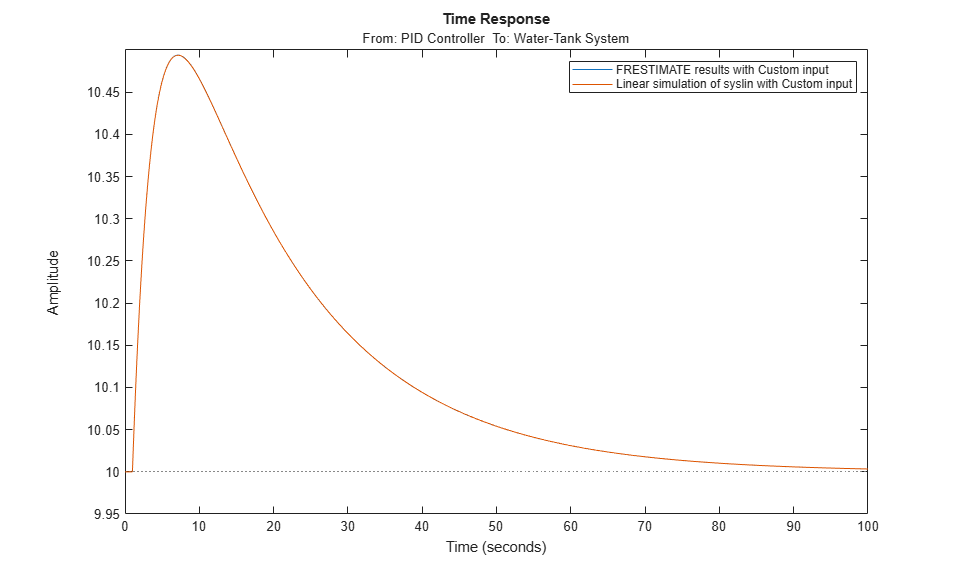Documentation

# frest.simCompare

Package: frest

Plot time-domain simulation of nonlinear and linear models

## Syntax

```frest.simCompare(simout,sys,input) frest.simCompare(simout,sys,input,x0) [y,t] = frest.simCompare(simout,sys,input) [y,t,x] = frest.simCompare(simout,sys,input,x0) ```

## Description

`frest.simCompare(simout,sys,input)` plots both

• Simulation output, `simout`, of the nonlinear Simulink model

You obtain the output from the `frestimate` command.

• Simulation output of the linear model `sys` for the input signal `input`

The linear simulation results are offset by the initial output values in the `simout` data.

`frest.simCompare(simout,sys,input,x0)` plots the frequency response simulation output and the simulation output of the linear model with initial state `x0`. Because you specify the initial state, the linear simulation result is not offset by the initial output values in the `simout` data.

`[y,t] = frest.simCompare(simout,sys,input)` returns the linear simulation output response `y` and the time vector `t` for the linear model `sys` with the input signal `input`. This syntax does not display a plot. The matrix `y` has as many rows as time samples `(length(t))` and as many columns as system outputs.

`[y,t,x] = frest.simCompare(simout,sys,input,x0)` also returns the state trajectory `x` for the linear state space model `sys` with initial state `x0`.

## Examples

collapse all

`frest.simCompare` lets you lets you examine the results of frequency response estimation in the time domain. You can compare the simulated model response to the response of a linear model of the system, such as one obtained by exact linearization.

Estimate the closed-loop response of the plant in the `watertank` model. First, open the model.

```model = 'watertank'; open_system(model); ```Define a linearization I/O set that specifies the plant, and find a steady-state operating point for estimation.

```io(1)=linio('watertank/PID Controller',1,'input'); io(2)=linio('watertank/Water-Tank System',1,'output'); watertank_spec = operspec(model); opOpts = findopOptions('DisplayReport','off'); op = findop(model,watertank_spec,opOpts); ```

Create an input signal for estimation. For this example, use a step input.

```input = frest.createStep('FinalTime',100); ```

Estimate the frequency response of the specified portion of the model, using the `simout` output argument to store the data generated during the estimation process. Also, linearize the model using the same I/O set and operating point.

```[sysest,simout] = frestimate(model,op,io,input); syslin = linearize(model,io,op); ```

Examine the time-domain responses of the linearized model and the Simulink model to the same input signal.

```frest.simCompare(simout,syslin,input) legend ```In this example, the responses are virtually identical.